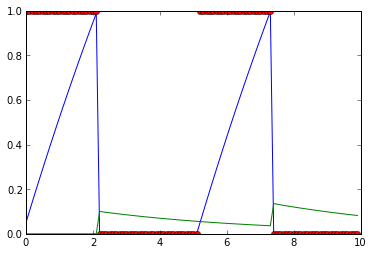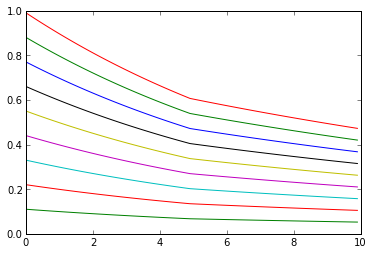# Brian²¶

In :
from brian2 import *


## Major changes for the user¶

### Arrays with unit support¶

In :
[1, 2, 3] * mV

Out:
$\left[\begin{smallmatrix}{}1.0 & 2.0 & 3.0\end{smallmatrix}\right]\,\mathrm{m}\,\mathrm{V}$
In :
mean(np.arange(12)*Hz)

Out:
$5.5\,\mathrm{Hz}$
In :
var(clip(randn(50)*nS, 0*nS, np.inf*nS))

Out:
$0.32033405871\,\mathrm{n}\,\mathrm{S}^{2}$
In :
G = NeuronGroup(10, 'dv/dt = -v / (10*ms) : volt')
G.v = -70*mV + randn(len(G))*3*mV
print mean(G.v[:])

-70.37843195 mV

• No more need to switch off unit-checking, units are not used during a run
• Everything is checked for correct units (including reset statements, threshold conditions, synaptic updates, ...)

### New refractoriness mechanism¶

In Brian 1, the default refractoriness mechanism clamped the value of the membrane potential during the refactory period. For other kinds of refractoriness, objects such as CustomRefractoriness had to be used. Brian² allows a more flexible formulation in the model equations and the refractory keyword argument.

In :
tau_v = 10*ms
tau_w = 5*ms
G = NeuronGroup(1, '''dv/dt = (5 - v + w) / tau_v : 1 (unless refractory)
dw/dt = -w / tau_w : 1''',
threshold='v>1', reset='v=0; w+=0.1', refractory=3*ms)
s_mon = StateMonitor(G, ['v', 'w', 'not_refractory'], record=True)
net = Network(G, s_mon)
net.run(10*ms)
plot(s_mon.t / ms, s_mon.v.T)
plot(s_mon.t / ms, s_mon.w.T)
_ = plot(s_mon.t / ms, s_mon.not_refractory.T, 'o')### Connection class is replaced by the Synapses class¶

In :
G1 = NeuronGroup(10, 'dv/dt = -v / (10*ms) : volt')
G2 = NeuronGroup(10, 'dv/dt = -v / (10*ms) : volt')
# Brian 1:
# C = Connection(G1, G2, 'v', delay=2*ms)
# C.connect_one_to_one(G1, G2, weight=1*mV)

S = Synapses(G1, G2, connect='i==j', pre='v+=1*mV', delay=2*ms)


The syntax for the Synapses class changed slightly compared to Brian 1:

In :
S = Synapses(G1, G2, 'w:volt', pre='v+=w')
# One-to-one connectivity
# In Brian1: S[:, :] = 'i==j'
S.connect('i==j')

# Full connectivity
# In Brian1: S[:, :] = True
S.connect(True)

# 10% connection probability
# In Brian1: S[:, :] = 0.1
S.connect(True, p=0.1)

# 2 synapses per connection
# In Brian1: S[:, :] = 2
S.connect(True, n=2)

# With a probability of 10%: 2 synapses per connection but only for one-to-one pairs
# S[:, :] = '(i==j) * (rand()<0.1) * 2'
S.connect('i==j', p=0.1, n=2)


### String-based indexing and string-based assignments¶

State variables of neurons or synapses can be accessed/set using strings. This is the recommended way of doing things!

In :
G = NeuronGroup(10, 'dv/dt = -v / (10*ms) : 1')
G.v = 1.0*np.arange(len(G)) / len(G)
print G.v[:]
G.v = '1.0*i / N' # i is the neuronal index, N the number of neurons
print G.v[:]

[ 0.   0.1  0.2  0.3  0.4  0.5  0.6  0.7  0.8  0.9]
[ 0.   0.1  0.2  0.3  0.4  0.5  0.6  0.7  0.8  0.9]

In :
G = NeuronGroup(10, 'dv/dt = -v / (10*ms) : 1')
S = Synapses(G, G, 'w:1', pre='v+=w', connect=True)
S.w['i!=j'] = 0.1
print S.w[:].reshape((10, 10))

[[ 0.   0.1  0.1  0.1  0.1  0.1  0.1  0.1  0.1  0.1]
[ 0.1  0.   0.1  0.1  0.1  0.1  0.1  0.1  0.1  0.1]
[ 0.1  0.1  0.   0.1  0.1  0.1  0.1  0.1  0.1  0.1]
[ 0.1  0.1  0.1  0.   0.1  0.1  0.1  0.1  0.1  0.1]
[ 0.1  0.1  0.1  0.1  0.   0.1  0.1  0.1  0.1  0.1]
[ 0.1  0.1  0.1  0.1  0.1  0.   0.1  0.1  0.1  0.1]
[ 0.1  0.1  0.1  0.1  0.1  0.1  0.   0.1  0.1  0.1]
[ 0.1  0.1  0.1  0.1  0.1  0.1  0.1  0.   0.1  0.1]
[ 0.1  0.1  0.1  0.1  0.1  0.1  0.1  0.1  0.   0.1]
[ 0.1  0.1  0.1  0.1  0.1  0.1  0.1  0.1  0.1  0. ]]


### Named objects¶

All Brian objects now have unique names (if no name is given, an automatic one is chosen)

• very useful for debugging (names are used in generated code)
• allows to get specific objects from a network
In :
# Automatic names
print G.name
print S.name

# Assigned name
E = NeuronGroup(10, 'dv/dt = -v/(10*ms) : 1', name='excitatory')
print E.name

neurongroup_4
synapses
excitatory

In :
def create_network():
G = NeuronGroup(10, 'dv/dt = -v/(10*ms) : 1', name='group')
mon = StateMonitor(G, 'v', record=True, name='monitor')
return Network(G, mon)

net = create_network()
print net['monitor']

<StateMonitor, recording ['v'] from group>


### More consistent/predictable handling of namespaces -- better support for multiple runs¶

Namespaces (mappings from names to values, e.g. "'v_th' means 0*mV") are handled quite differently from Brian 1:

• A namespace can be defined individually for a Brian object (NeuronGroup, Synapses). It does not have to be complete at the time of the object creation, it can be later filled in (via the namespace attribute) and only has to be complete at the start of a run
• For objects that did not specify an individual namespace, the namespace is filled with the namespace at the point of the run function (either from an explicit namespace argument, or from the surrounding "implicit" namespace)
• Namespaces can change between runs
• Note: For simple, "flat" scripts (e.g. most of the Brian examples), nothing changes
In :
# Explicit namespace
G = NeuronGroup(10, 'dv/dt = -v / tau : 1', namespace={'tau': 10*ms})
G.v = linspace(0, 1, len(G))
mon = StateMonitor(G, 'v', record=True)
net = Network(G, mon)
net.run(5*ms)
G.namespace['tau'] = 20*ms
net.run(5*ms)

_ = plot(mon.t / ms, mon.v.T)In :
# Implicit namespace
G = NeuronGroup(10, 'dv/dt = -v / tau : 1')
tau = 10*ms  # does not need to be defined before!
G.v = linspace(0, 1, len(G))
mon = StateMonitor(G, 'v', record=True)
net = Network(G, mon)
net.run(5*ms)
tau = 20*ms  # this would have no effect in Brian 1!
net.run(5*ms)

_ = plot(mon.t / ms, mon.v.T)#### New system for explicit numerical integration methods¶

Explicit numerical integration methods can be specified using mathematical notation

In :
forward_euler = ExplicitStateUpdater('x_new = x + dt * f(x, t)')
forward_euler

Out:
\begin{equation} x_{t+1} = dt f{\left (x,t \right )} + x \end{equation}
In :
print forward_euler(Equations('''dv/dt = (-v + w)/ tau_v : 1
dw/dt = -w / tau_w : 1'''))

_w = -dt*w/tau_w + w
_v = dt*(-v + w)/tau_v + v
w = _w
v = _v

• Stochastic equations are handled properly, Euler integration can used for additive noise, the derivative-free Milstein method for multiplicative noise
• The state updater system is easily extendable, no core code has to change when a new state updater is added

### Stochastic integration¶

Single noise variable:

In :
eqs = Equations('dv/dt = -v / tau + sigma*xi/tau**0.5 : 1')
print euler(eqs)

xi = dt**.5 * randn()
_v = -dt*v/tau + v + sigma*tau**(-0.5)*xi
v = _v


Independent noise variables (names are arbitrary, one can also use xi_alpha, xi_beta, it only has to start with xi_...)

In :
eqs = Equations('''dv/dt = -v / tau + sigma*xi_1/tau**0.5 : 1
dw/dt = -w / tau + sigma*xi_2/tau**0.5 : 1''') # simply using 'xi' raises an error
print euler(eqs)

xi_1 = dt**.5 * randn()
xi_2 = dt**.5 * randn()
_w = -dt*w/tau + w + sigma*tau**(-0.5)*xi_2
_v = -dt*v/tau + v + sigma*tau**(-0.5)*xi_1
w = _w
v = _v


Shared noise variables:

In :
eqs = Equations('''dv/dt = -v / tau + sigma*xi_shared/tau**0.5 : 1
dw/dt = -w / tau + sigma*xi_shared/tau**0.5 : 1''')
print euler(eqs)

xi_shared = dt**.5 * randn()
_w = -dt*w/tau + w + sigma*tau**(-0.5)*xi_shared
_v = -dt*v/tau + v + sigma*tau**(-0.5)*xi_shared
w = _w
v = _v


### Further new features¶

#### "Rich display" in ipython notebooks¶

In :
eqs = Equations('''dv/dt = (g_L*(E_L - v) + g_s*(E_s - v))/tau_m : volt
dg_s/dt = -g_s/tau_s : siemens''')
eqs

Out:
\begin{align*}\frac{\mathrm{d}g_{s}}{\mathrm{d}t} &= - \frac{g_{s}}{\tau_{s}} && \text{(unit: $\mathrm{S}$)}\\ \frac{\mathrm{d}v}{\mathrm{d}t} &= \frac{1}{\tau_{m}} \left(g_{L} \left(E_{L} - v\right) + g_{s} \left(E_{s} - v\right)\right) && \text{(unit: $\mathrm{V}$)}\end{align*}

#### New preference system¶

Accessable using either attribute or dictionary access

In :
print brian_prefs.core.default_scalar_dtype
print brian_prefs['core.default_scalar_dtype']

<type 'numpy.float64'>
<type 'numpy.float64'>

• Default preference values are stored in a file in the brian installation directory, can be overwritten using a file in the user's home directory or in the simulation directory
• Extensions to Brian can create and document their own preferences, they are accessible via the common system

#### New logging system¶

By default, a debug log is written to the temp directory, will normally be deleted after a run but will be kept if an uncaught exception occurs.

#### New documentation system¶

• Brian now uses it's own extension of the numpydoc sphinx extension, allowing for docstrings that are well readable in source code and generate well-readable HTML documentation.
• Reference documentation for all Brian classes is automatically created and kept up-to-date.

## Current state of Brian 2¶

Useable for curious users and up for testing, download it from github: https://github.com/brian-team/brian2

You can directly download and install it with pip: pip install -e git+https://github.com/brian-team/brian2

What is missing?

• Handling of subgroups, linked variables between NeuronGroups
• dynamic connections (provided by the old Connections object)
• many convenience classes: TimedArray, PoissonInput, PopulationRateMonitor, etc.
• Support for other codegeneration targets than Python or C++ (e.g. CUDA)
• User-documentation (beyond the class reference)
• Developer documentation that accurately reflects the current state everywhere :)
• A lot of cleaning up/polishing

What's next?

• Alpha version soon
• Feature-complete Beta version after the summer
• First release at the end of the year